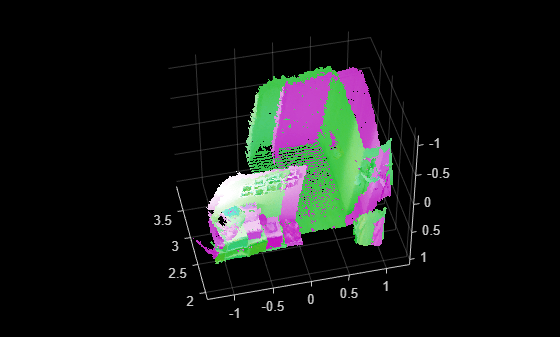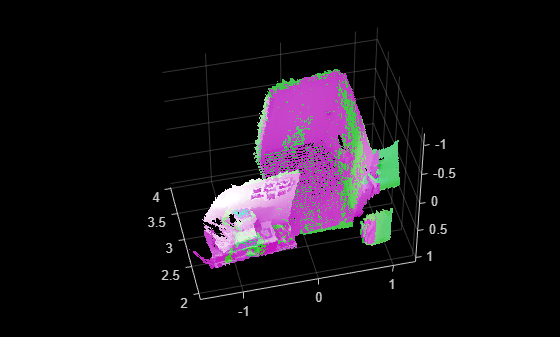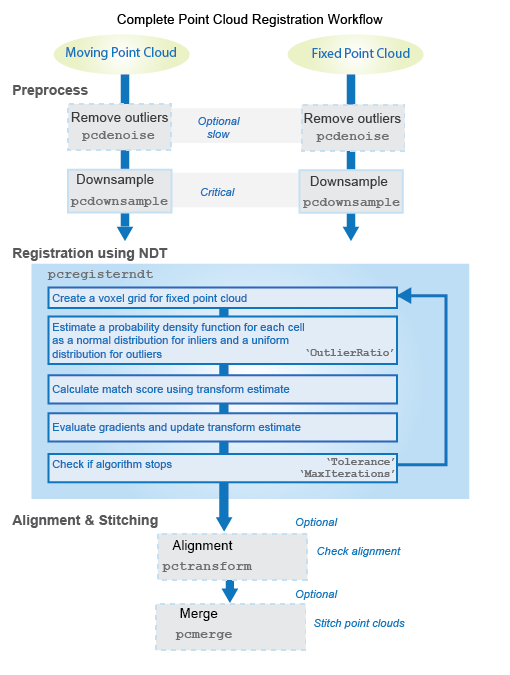# pcregisterndt

Register two point clouds using NDT algorithm

## Syntax

``tform = pcregisterndt(moving,fixed,gridStep)``
``[tform,movingReg] = pcregisterndt(moving,fixed,gridStep)``
``[___,rmse] = pcregisterndt(moving,fixed,gridStep)``
``[___] = pcregisterndt(moving,fixed,gridStep,Name=Value)``

## Description

example

````tform = pcregisterndt(moving,fixed,gridStep)` returns the rigid transformation that registers the moving point cloud with the fixed point cloud. The point clouds are voxelized into cubes of size `gridStep`.The registration algorithm is based on the normal-distributions transform (NDT) algorithm  . Best performance of this iterative process requires adjusting properties for your data. To improve accuracy and efficiency of registration, consider downsampling the point clouds by using `pcdownsample` before using `pcregisterndt`.```
````[tform,movingReg] = pcregisterndt(moving,fixed,gridStep)` also returns the transformed point cloud that aligns with the fixed point cloud.```
````[___,rmse] = pcregisterndt(moving,fixed,gridStep)` also returns the root mean square error of the Euclidean distance between the aligned point clouds, using any of the preceding syntaxes.```
````[___] = pcregisterndt(moving,fixed,gridStep,Name=Value)` specifies options using one or more name-value arguments in addition to any combination of arguments from previous syntaxes. For example, `MaxIterations=20` stops the NDT algorithm after 20 iterations. ```

## Examples

collapse all

```ld = load('livingRoom.mat'); moving = ld.livingRoomData{1}; fixed = ld.livingRoomData{2}; pcshowpair(moving,fixed,'VerticalAxis','Y','VerticalAxisDir','Down')```To improve the efficiency and accuracy of the NDT registration algorithm, downsample the moving point cloud.

`movingDownsampled = pcdownsample(moving,'gridAverage',0.1);`

Voxelize the point cloud into cubes of sidelength 0.5. Apply the rigid registration using the NDT algorithm.

```gridStep = 0.5; tform = pcregisterndt(movingDownsampled,fixed,gridStep);```

Visualize the alignment.

```movingReg = pctransform(moving,tform); pcshowpair(movingReg,fixed,'VerticalAxis','Y','VerticalAxisDir','Down')```## Input Arguments

collapse all

Moving point cloud, specified as a `pointCloud` object.

Fixed point cloud, specified as a `pointCloud` object.

Size of the 3-D cube that voxelizes the fixed point cloud, specified as a positive scalar.

Data Types: `single` | `double`

### Name-Value Arguments

Specify optional pairs of arguments as `Name1=Value1,...,NameN=ValueN`, where `Name` is the argument name and `Value` is the corresponding value. Name-value arguments must appear after other arguments, but the order of the pairs does not matter.

Example: `MaxIterations=20` stops the NDT algorithm after 20 iterations.

Initial rigid transformation, specified as a `rigid3d` object. Set the initial transformation to a coarse estimate. If you do not provide a course or initial estimate, the function uses a `rigid3d` object that contains a translation that moves the center of the moving points to the center of the fixed points.

Expected percentage of outliers with respect to a normal distribution, specified as a scalar in the range [0, 1). The NDT algorithm assumes a point is generated by a mixture of a normal distribution for inliers and a uniform distribution for outliers. A larger value of '`OutlierRatio`' reduces the influence of outliers.

Data Types: `single` | `double`

Maximum number of iterations before NDT stops, specified as a nonnegative integer.

Data Types: `single` | `double`

Tolerance between consecutive NDT iterations, specified as a 2-element vector with nonnegative values. The vector, [Tdiff Rdiff], represents the tolerance of absolute difference in translation and rotation estimated in consecutive NDT iterations. Tdiff measures the Euclidean distance between two translation vectors. Rdiff measures the angular difference in degrees. The algorithm stops when the difference between estimated rigid transformations in the most recent consecutive iterations falls below the specified tolerance value.

Data Types: `single` | `double`

Display progress information, specified as a numeric or logical `0` (`false`) or `1` (`true`). To display the progress information, set `Verbose` to `true`.

## Output Arguments

collapse all

Rigid transformation, returned as a `rigid3d` object. `tform` describes the rigid 3-D transformation that registers the moving point cloud, `moving`, to the fixed point cloud, `fixed`.

Transformed point cloud, returned as a `pointCloud` object. The transformed point cloud is aligned with the fixed point cloud, `fixed`.

Root mean square error, returned as a positive number. `rmse` is the Euclidean distance between the aligned point clouds. A low `rmse` value indicates a more accurate registration.

## Algorithms

collapse all### NDT Registration Overview

The NDT registration is based on normal distributions rather than other types of registration which match points or lines in order to find the transformation between two point clouds. The NDT approach uses statistical models with 3-D boxes, called voxels.

To register two point clouds, a moving point cloud and a fixed point cloud, using the NDT approach, the algorithm performs the following:

1. Computes the normal distributions for the fixed point cloud by dividing the area covered by the point cloud scan into 3-D boxes of constant size, referred to as "voxels". Each voxel contains a set of points. The algorithm computes the mean and covariance matrix for the points in each voxel.

2. Starting with an initial guess of the transformation, the algorithm aligns the moving point cloud to the fixed point cloud. It then finds the sum of the statistical likelihood of each aligned point to be located in the voxel that surrounds the point (in the moving point cloud), based on the normal distribution of the fixed point cloud.

3. To improve the registration, the algorithm maximizes the probability score of the moving point cloud on the normal distributions of the fixed point cloud. This is done by iteratively optimizing angular and translational estimations.

4. Repeats the alignment of the moving point cloud with the fixed piont cloud using the new transformation from the previous step and then repeats the optimization.

5. The algorithm stops once the maximum iterations or the tolerance parameters, are met. The tolerance is a measure of how much the angular and translational estimations change from one iteration to another.

 Biber, P., and W. Straßer. “The Normal Distributions Transform: A New Approach to Laser Scan Matching.” Proceedings of IEEE/RSJ International Conference on Intelligent Robots and Systems (IROS). Las Vegas, NV. Vol. 3, November 2003, pp. 2743–2748.

 Magnusson, M. “The Three-Dimensional Normal-Distributions Transform — an Efficient Representation for Registration, Surface Analysis, and Loop Detection.” Ph.D. Thesis. Örebro University, Örebro, Sweden, 2013.